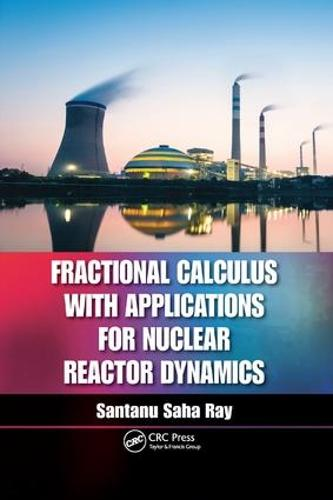•# Fractional Calculus with Applications for Nuclear Reactor Dynamics (Paperback)

(author)
£77.99
Paperback 240 Pages / Published: 26/07/2017
• We can order this

Usually dispatched within 3 weeks

Introduces Novel Applications for Solving Neutron Transport Equations

While deemed nonessential in the past, fractional calculus is now gaining momentum in the science and engineering community. Various disciplines have discovered that realistic models of physical phenomenon can be achieved with fractional calculus and are using them in numerous ways. Since fractional calculus represents a reactor more closely than classical integer order calculus, Fractional Calculus with Applications for Nuclear Reactor Dynamics focuses on the application of fractional calculus to describe the physical behavior of nuclear reactors. It applies fractional calculus to incorporate the mathematical methods used to analyze the diffusion theory model of neutron transport and explains the role of neutron transport in reactor theory.

The author discusses fractional calculus and the numerical solution for fractional neutron point kinetic equation (FNPKE), introduces the technique for efficient and accurate numerical computation for FNPKE with different values of reactivity, and analyzes the fractional neutron point kinetic (FNPK) model for the dynamic behavior of neutron motion. The book begins with an overview of nuclear reactors, explains how nuclear energy is extracted from reactors, and explores the behavior of neutron density using reactivity functions. It also demonstrates the applicability of the Haar wavelet method and introduces the neutron diffusion concept to aid readers in understanding the complex behavior of average neutron motion.

This text:

Applies the effective analytical and numerical methods to obtain the solution for the NDEDetermines the numerical solution for one-group delayed neutron FNPKE by the explicit finite difference methodProvides the numerical solution for classical as well as fractional neutron point kinetic equationsProposes the Haar wavelet operational method (HWOM) to obtain the numerical approximate solution of the neutron point kinetic equation, and more

Fractional Calculus with Applications for Nuclear Reactor Dynamics thoroughly and systematically presents the concepts of fractional calculus and emphasizes the relevance of its application to the nuclear reactor.

Publisher: Taylor & Francis Ltd
ISBN: 9781138893238
Number of pages: 240
Weight: 454 g
Dimensions: 234 x 156 mm

MEDIA REVIEWS

"The textbook covers a wide range of models and methods for neutron transport and will be a welcome addition to many undergraduate and graduate courses. Graduate students working in nuclear engineering neutron transport area will appreciate the details of the methods, and will find this book useful to guide their research."
-Igor A. Bolotnov, Department of Nuclear Engineering, North Carolina State University

"I have read the two sample chapters provided with me. I found no irregulaity in these two chapters. The table of content is absolutely ideal. This book focuses on the application of fractional calculus to describe the physical behavior of nuclear reactors. The sample chapters reflect the concept of the subject fairly.

In my opinion this book will be indispensable in the field of nuclear reactor science and engineering."

-Dr. Rasajit Kumar Bera, M. Sc. (Applied Maths., Gold Medalist), Ph. D.(Sc.), Retired Professor & Head of the Department of Science, National Institute of Technical Teacher's Training and Research (Eastern Region), Block-FC, Sector-III, Salt Lake City, Kolkata-700106, India